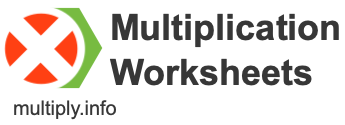Multiplication WorksheetsHere you can download and/or print thousands of multiplication worksheets for free. You have permission to print and use as many copies of our multiplication worksheets as you'd like, as long as you don't alter or remove any references to multiply.info.

We have different options to accommodate a variety of skill levels and grades. Maybe a student needs extra practice on a certain multiplication table, or maybe a student wants a challenge. Regardless of the situation, we have multiplication worksheets for all scenarios.

Single
Create a times table worksheet for just one number. If you choose "3", for example, the worksheet will only contain problems related to the multiplication table of 3.

Double
Create a times table worksheet for two numbers. If you choose "2 & 3", for example, the worksheet will only contain problems related to the multiplication table of 2 and the multiplication table of 3.

Range
Create a times table worksheet for a range of numbers. If you choose "1 - 4", for example, the worksheet will only contain problems related to the multiplication tables of 1, 2, 3, and 4.

Less or More
Restrict small or large numbers from the multiplication tables. If you choose "4 or less", for example, the worksheet will only contain numbers that are less than or equal to 4.

Digits
Choose how many digits of each factor (multiplicand and multiplier) you want in the multiplication problem. For example, choosing "aa × b" will generate problems such as "12 times 3".

Mixed
A multiplication problem has three parts: multiplicand, multiplier, and product (or factor, factor, and product). Here you can create worksheets where the task is to calculate the missing part. Any of the three parts could be missing, so the worksheet contains a mix of problems.

Multiplication Worksheet Solutions
The solutions to all of our multiplication worksheets are free and available online for teachers, educators and students. Go here to find answers to a specific worksheet by entering the worksheet number.

Reprint Worksheets